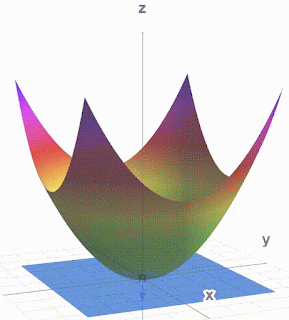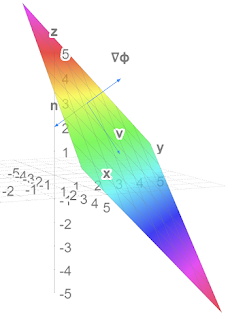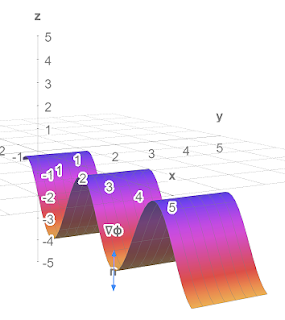## Monday, 22 November 2021

### 3D-grapher at math3d.org

I was practicing my tensor analysis and calculated a normal vector to a surface. I wanted to see it looked orthogonal to the surface. It didn't really. So I made a tangent plane to the surface. The normal vector still looks a bit un-orthogonal but it passes all the mathematical tests.Paraboloid, Normal vector and tangent plane $\phi_2\left(\vec{x}\right)=\left(x^1\right)^2+\left(x^2\right)^2-x^3=0$

You can play with this at and stop it from moving.
I also used Debut Video Capture to make a .avi from math3d and then cloudconvert.com to make the .gif. It is simpler than ezgif.com.

Other surfaces and vectors:Plane and normal vectors (not v)Play at https://www.math3d.org/fq5CtGqYa$\phi_1\left(\vec{x}\right)=x^1+x^2+x^3=5$Sine wave with normal vectors Play at https://www.math3d.org/D8xf0gZMT$\phi_3\left(\vec{x}\right)=x^3+r_0\cos{\left(kx^1\right)}=-4$

Lots more tools on Physics Forums at